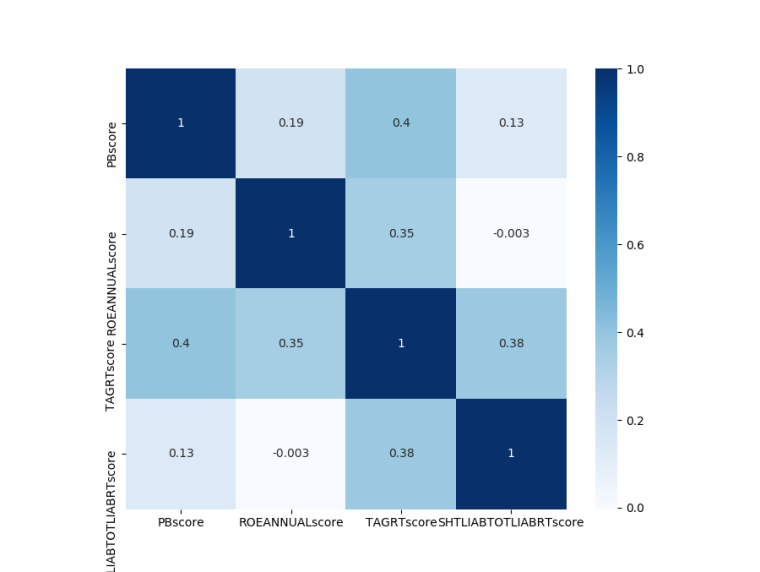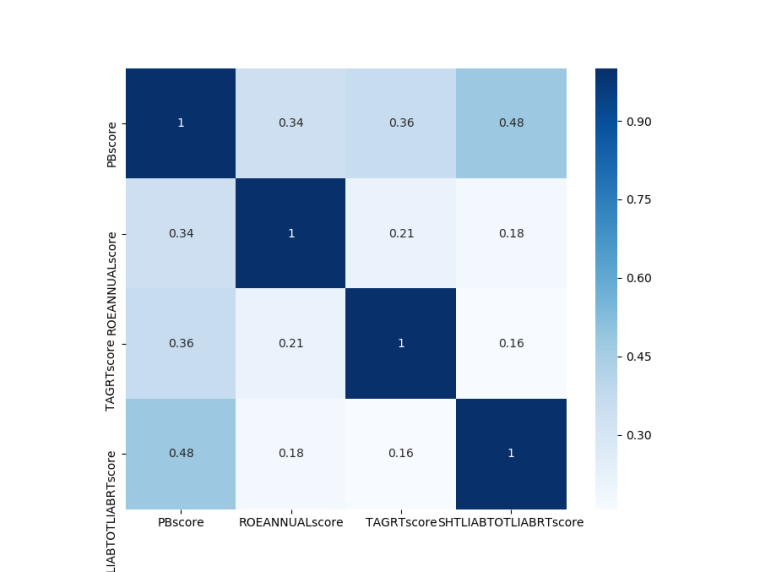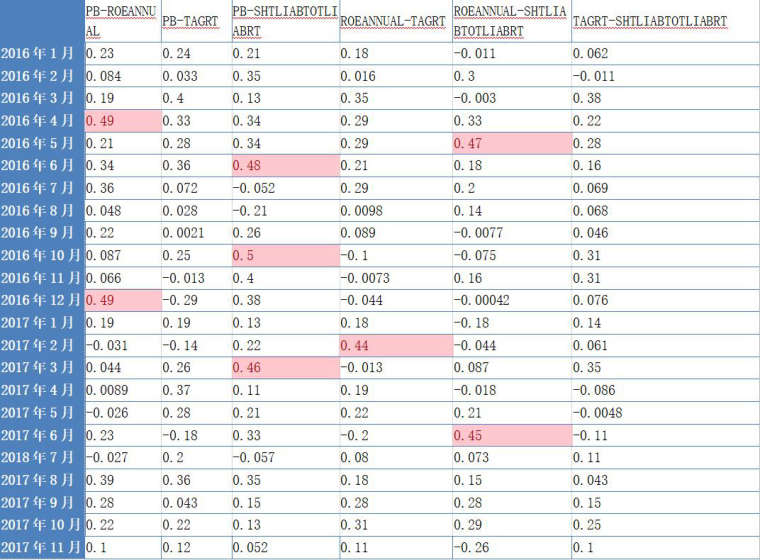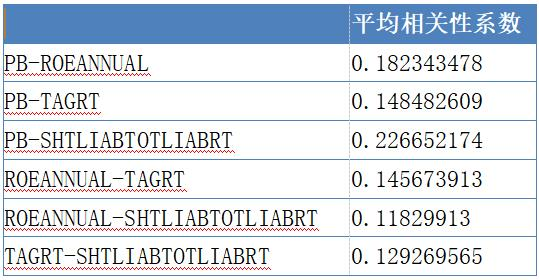掘金小Q 【 论坛管理员 】 发表在策略分享 2018-08-08 17:59:57

3724
0
0

1、分别按不同因子的大小进行排序，讲股票分为n个组合进行打分，分值与该组合在整个模型形成期的收益相关，收益越大，分值越高。分值赋给每月该组合内的所有个股。

1,2,3,4,5,6,7,8,9,10。如果组合1的收益大于组合10，那么正好是反过来 10,9,8,7,6,5,4,3,2,1。

2、按月计算个股的不同因子得分间的相关性矩阵。

3、在计算完每月因子得分相关性矩阵后，计算整个样本期内相关性矩阵的平均值。

4、设定一个得分相关性阀值，将得分相关性平均值矩阵中大于该阀值的元素所对应的因子只保留与其他因子相关性较小、有效性更强的因子，而其它因子则作为冗余因子剔除。1、获取每个月的首个交易日，月初获取每只股票的因子数据及当月收益率，根据因子数值排序，将股票分为10组，根据平均收益为股票组合打分，分值赋予对应组合中所有股票。

2、分别测试股票关于四种因子的分值，根据每月结果，计算相关性系数矩阵。

3、计算整个测试周期的相关性系数平均值。

4、设定阈值，剔除冗余因子。2016年3月2016年4月2016年6月2016年10月2016年12月2017年6月from __future__ import print_function, absolute_import, unicode_literals
import seaborn as sns
import pandas as pd
from gm.api import *
import matplotlib.pyplot as plt
set_token('xxxxxxxxxxxx')

#本策略基于掘金量化交易平台 网址：www.myquant.cn

#相关性矩阵热力图
def cor(df):
dfData = df.corr()
plt.subplots(figsize=(9, 9)) # 设置画面大小
sns.heatmap(dfData, annot=True, vmax=1, square=True, cmap="Blues")
plt.savefig('./BluesStateRelation.png')
plt.show()

#获取每月第一个交易日
date=[]
for i in time:
n=time.index(i)
if i[5:7]!=time[n-1][5:7]:
date.append(i)

#测试因子间相关性
data=pd.DataFrame()
fin=pd.DataFrame()
a=0
for factor in ['PB','ROEANNUAL','TAGRT','SHTLIABTOTLIABRT']:
if n ==23:
break
# 获取上一个交易日
nextmoth=date[n+1]
# 获取上证50成份股
stock300 = get_history_constituents(index='SHSE.000016', start_date=last_day,
end_date=last_day)['constituents'].keys()
# 获取当天有交易的股票
not_suspended_symbols = [item['symbol'] for item in not_suspended_info if not item['is_suspended']]
#获取因子数据
if factor=='PB':
filter='', order_by='-' + factor, df=True)
else:
fin = get_fundamentals(table='deriv_finance_indicator', symbols=not_suspended_symbols,
filter='', order_by='-'+factor, df=True)
long=len(fin)
fin=fin.fillna(0)
portfolio=[]

#获取股票收益率
for i in fin.symbol:
try :
last=history(symbol=i, frequency='1d', start_time=nextmoth, end_time=nextmoth, fields='close', skip_suspended=True,
except:
portfolio.append(0)
continue
portfolio.append((last - pre) / pre)
#根据收益率对股票进行打分
portfolio_2=[(sum(portfolio[0:4]))/5]*5+[(sum(portfolio[5:9]))/5]*5+[(sum(portfolio[10:14]))/5]*5+[(sum(portfolio[15:19]))/5]*5+[(sum(portfolio[20:24]))/5]*5+[(sum(portfolio[25:29]))/5]*5+[(sum(portfolio[30:34]))/5]*5+[(sum(portfolio[35:39]))/5]*5+[(sum(portfolio[40:44]))/5]*5+[(sum(portfolio[45-long:0]))/5]*(long-45)
fin['portfolio_2'] = portfolio_2
fin=fin.sort_values(by = 'portfolio_2',axis = 0,ascending = False)
score=*5+*5+*5+*5+*5+*5+*5+*5+*5+*(long-45)
fin[factor + 'score'] =score
del fin['pub_date'],fin['end_date'],fin['portfolio_2'],fin[factor]
a=a+1
#获取关于四个因子下，每个股票所获得的分数值
if a==1:
data=fin
else:
data = pd.merge(data, fin, how='right', on='symbol')
#统计分析
del data['symbol']
cor(data)
print(data.corr())



Looks like your connection to 掘金量化社区 - 量化交易者的交流社区 was lost, please wait while we try to reconnect.

• 提问
遇到问题？到社区提问，我们为您解答.
• 商业
咨询
有商业合作意向？请发邮件联系我们.
• QQ
加入掘金量化QQ群
• 公众号
关注掘金量化微信公众号
• TOP
返回顶部